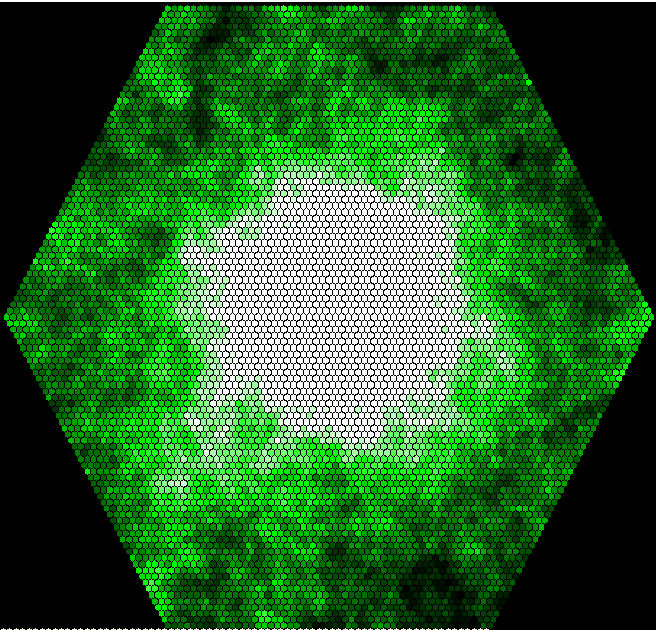# Hexagonal grid

## Animal movement and interaction with their environment

ModelId:
antsworld
SimileVersion:
5.9

In this model, a population of animals (called ants) is created and the individuals move around at random. The area in which they move is represented by a grid of hexagons.

An association model is created to relate each individual to the hexagon it currently occupies. The technique of one-sided enumeration is used to make this association; it is vital that it happens efficiently, because it changes each time step. In this case the position of each ant is used to determine the index of the submodel instance for the hexagon containing it.

Equations:

Equations in antsworld

Variable n
n = int(sqrt(size(World)))

Variable neighbour offsets a
neighbour offsets a = [0,1,1,0,-1,-1]

Variable neighbour offsets b
neighbour offsets b = [1,0,-1,-1,0,1]

Variable neighbour offsets c
neighbour offsets c = [-1,-1,0,1,1,0]

Equations in Ants

Immigration im1
im1 = 0.1

Variable Pheromone output
Pheromone output = 1

Variable my body size
my body size = 2

Variable my direction
my direction = ceil(rand_var(0,6))

Variable my space
my space = infront=element(last([neighbour_spaces]),my_direction),if time()==init_time() then 5102 elseif infront>0 then infront else prev(1)
Where:
my_direction=my direction
[neighbour_spaces]=neighbour spaces

Variable neighbour spaces
neighbour spaces = [neighbours_at]
Where:
[neighbours_at]= ../location/neighbours (to Ants in at)

Variable x
x = x_at
Where:
x_at= ../location/x (to Ants in at)

Variable y
y = y_at
Where:
y_at= ../location/y (to Ants in at)

Equations in location

Condition cond1
cond1 = index(1) is my_space_at
Where:
my_space_at= ../Ants/my space (from Ants in at)

Variable Pheromone
Pheromone = Pheromone_output_at
Where:
Pheromone_output_at= ../Ants/Pheromone output (from Ants in at)

Variable neighbours
neighbours = [their_ids_has]
Where:

[their_ids_has]= ../World/neighbours/their ids (from World in has)

Variable x
x = x_has
Where:
x_has= ../World/x (from World in has)

Variable y
y = y_has
Where:
y_has= ../World/y (from World in has)

Equations in World

Compartment Pheromone
Initial value = 0
Rate of change = + Addition

Where:
{Pheromone_has}= ../location/Pheromone (to World in has)

Variable a
a = ceil(index(1)/n)-ceil(n/2)
Where:
n= ../n

Variable b
b = index(1)-n*a-ceil(n*n/2)
Where:
n= ../n

Variable c
c = -a-b

Variable my id
my id = index(1)

Variable x
x = 50+(a-c)* 0.866

Variable y
y = 50+b* 1.5

Equations in borders

Variable x
x = off=sqrt(3/4),x+element([0,off,off,0,-off,-off],index(1))
Where:
x= ../x

Variable y
y = y+element([1, 0.5, -0.5,-1, -0.5, 0.5],index(1))
Where:
y= ../y

Equations in neighbours

Variable a
a = a+element([neighbour_offsets_a],index(1))
Where:
a= ../a
[neighbour_offsets_a]= ../../neighbour offsets a

Variable b
b = b+element([neighbour_offsets_b],index(1))
Where:
b= ../b
[neighbour_offsets_b]= ../../neighbour offsets b

Variable c
c = c+element([neighbour_offsets_c],index(1))
Where:
c= ../c
[neighbour_offsets_c]= ../../neighbour offsets c

Variable their ids
their ids = if all([a,b,c]> - (n/2)) and all([a,b,c]

Results:

This image shows the distribution of pheromone over the grid are after 3000 time units: